• matlab作概率密度函数很简单，但是本人学的python，因此想用python作图，从txt文本中读取数据，然后用python概率密度函数图像，
• 主要介绍了python 计算概率密度、累计分布、逆函数的例子,具有很好的参考价值，希望对大家有所帮助。一起跟随小编过来看看吧
• 今天小编就为大家分享一篇python高斯分布概率密度函数的使用详解，具有很好的参考价值，希望对大家有所帮助。一起跟随小编过来看看吧
• 今天小编就为大家分享一篇python实现beta分布概率密度函数的方法，具有很好的参考价值，希望对大家有所帮助。一起跟随小编过来看看吧
• 二维高斯分布概率密度函数数据集实战优化坐标轴与图像优化图像再次优化 概率密度函数 大家肯定都有听说过正态分布，其实正态分布只是概率密度分布的一种，正态分布的概率密度函数均值为μ ，标准差σ是高斯函数的一...
• pdf：连续随机分布的概率密度函数 pmf：离散随机分布的概率密度函数 cdf：累计分布函数 百分位函数（累计分布函数的逆函数） 生存函数的逆函数（1 - cdf 的逆函数） 函数里面不仅能跟一个数据，还能跟一个数组。...
计算概率分布的相关参数时，一般使用 scipy 包，常用的函数包括以下几个：
pdf：连续随机分布的概率密度函数pmf：离散随机分布的概率密度函数cdf：累计分布函数ppf: 百分位函数（累计分布函数的逆函数）lsf: 生存函数的逆函数（1 - cdf 的逆函数）
函数里面不仅能跟一个数据，还能跟一个数组。下面用正态分布举例说明：
>>> import scipy.stats as st

>>> st.norm.cdf(0) # 标准正态分布在 0 处的累计分布概率值
0.5

>>> st.norm.cdf([-1, 0, 1])# 标准正态分布分别在 -1， 0， 1 处的累计分布概率值
array([0.15865525, 0.5, 0.84134475])

>>> st.norm.pdf(0) # 标准正态分布在 0 处的概率密度值
0.3989422804014327

>>> st.norm.ppf(0.975)# 标准正态分布在 0.975 处的逆函数值
1.959963984540054

>>> st.norm.lsf(0.975)# 标准正态分布在 0.025 处的生存函数的逆函数值
1.959963984540054

对于非标准正态分布，通过更改参数 loc 与 scale 来改变均值与标准差：
>>> st.norm.cdf(0, loc=2, scale=1) # 均值为 2，标准差为 1 的正态分布在 0 处的累计分布概率值
0.022750131948179195

对于其他随机分布，可能更改的参数不一样，具体需要查官方文档。下面我们举一些常用分布的例子：
>>> st.binom.pmf(4, n=100, p=0.05) # 参数值 n=100, p=0.05 的二项分布在 4 处的概率密度值
0.17814264156968956

>>> st.geom.pmf(4, p=0.05) # 参数值 p=0.05 的几何分布在 4 处的概率密度值
0.04286875

>>> st.poisson.pmf(2, mu=3) # 参数值 mu=3 的泊松分布在 2 处的概率密度值
0.22404180765538775

>>> st.chi2.ppf(0.95, df=10) # 自由度为 10 的卡方分布在 0.95 处的逆函数值
18.307038053275146

>>> st.t.ppf(0.975, df=10) # 自由度为 10 的 t 分布在 0.975 处的逆函数值
2.2281388519649385

>>> st.f.ppf(0.95, dfn=2, dfd=12) # 自由度为 2, 12 的 F 分布在 0.95 处的逆函数值
3.8852938346523933

展开全文scipy
• python 服从正态分布下概率密度函数和累积密度函数 服从正太分布下,概率密度函数公式 公式解释: f(x): 是某样本(样本以数值形式表现)为某数值时发生的概率 0<f(x)<1 x: 是随机抽样的数值,取值范围从负...
python 服从正态分布下概率密度函数
服从正太分布下,概率密度函数公式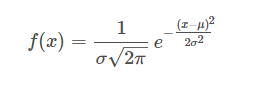公式解释: f(x): 是某样本(样本以数值形式表现)为某数值时发生的概率 0<f(x)<1
x: 是随机抽样的数值,取值范围从负无穷大到正正无穷大 e: 是自然数 σ: 是样本的标准差 μ:是样本的算术平均值(也叫均值)
对服从正太分布下,概率密度函数的理解: (1) 当自变量x=μ时,f(x)取的最大值, 最大值=f(x=μ) 即:当自变量取值为均值时,发生的概率最大(或者说:发生概率最大的数是均值)
(2) 概率密度函数的图像(曲线图像)关于x=μ对称
(3) 标准差σ越大, 则图像峰值(峰值也就是概率最大值,即:峰值=f(x=μ))越小
(4) 概率最小值趋近于0
应用python绘制图像
"""
绘制正太分布函数曲线图

"""
import matplotlib.pyplot as plt
import math
import numpy as np
import matplotlib

matplotlib.rcParams['axes.unicode_minus']=False#解决保存图像时负号'-'显示为方块的问题
plt.rcParams['font.sans-serif'] = ['SimHei'] # 指定默认字体

x = np.linspace(-10,30,num=1000)  # x轴的取值范围
std1 = 1 # 定义标准差, 并输入标准差
mean1 = 10  # 定义均值,并输入均值
fx1 = 1 / (std1 * pow(2 * math.pi, 0.5)) * np.exp(-((x - mean1) ** 2) / (2 * std1 ** 2))  # 概率密度函数公式

std2 = 2
mean2 = 10
fx2 = 1 / (std2 * pow(2 * math.pi, 0.5)) * np.exp(-((x - mean2) ** 2) / (2 * std2 ** 2))  # 概率密度函数公式

std3 = 4
mean3= 10
fx3 = 1 / (std3 * pow(2 * math.pi, 0.5)) * np.exp(-((x - mean3) ** 2) / (2 * std3 ** 2))  # 概率密度函数公式

std4 = 8
mean4 = 10
fx4 = 1 / (std4 * pow(2 * math.pi, 0.5)) * np.exp(-((x - mean4) ** 2) / (2 * std4 ** 2))  # 概率密度函数公式

# 多条曲线在同一张图上进行对比
plt.plot(x, fx1,label = 'std1 = 1')  # 绘制概率密度函数图像
plt.plot(x,fx2,label = 'std2 = 2')
plt.plot(x,fx3,label = 'std3 = 4')
plt.plot(x,fx4,label = 'std4 = 8')
plt.legend() # 显示标签 label
plt.xlabel("数值")
plt.ylabel('数值的概率')
plt.title('服从正太分布的概率密度图')
plt.show()  # 显示图像

显示图像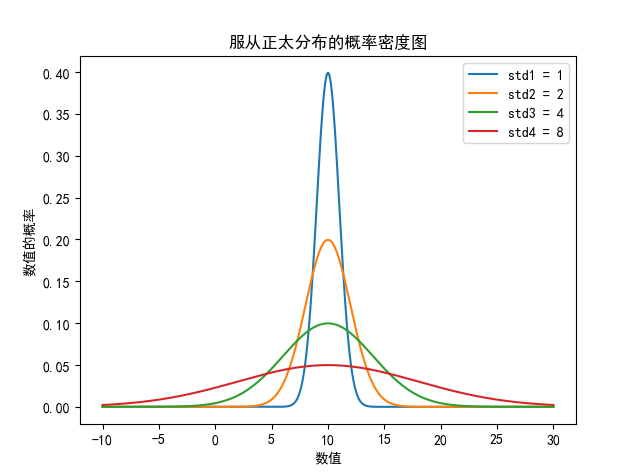由图像可知道: 标准差std 越大, 则峰值越小.(代码设置了均值相同)
稍微修改均值和标准差后,重新绘图, 如下:
import matplotlib.pyplot as plt
import math
import numpy as np
import matplotlib

matplotlib.rcParams['axes.unicode_minus']=False#解决保存图像时负号'-'显示为方块的问题
plt.rcParams['font.sans-serif'] = ['SimHei'] # 指定默认字体

x = np.linspace(-10,30,num=1000)  # x轴的取值范围
std1 = 3 # 定义标准差, 并输入标准差
mean1 = 6  # 定义均值,并输入均值
fx1 = 1 / (std1 * pow(2 * math.pi, 0.5)) * np.exp(-((x - mean1) ** 2) / (2 * std1 ** 2))  # 概率密度函数公式

std2 = 3
mean2 = 10
fx2 = 1 / (std2 * pow(2 * math.pi, 0.5)) * np.exp(-((x - mean2) ** 2) / (2 * std2 ** 2))  # 概率密度函数公式

std3 = 3
mean3= 12
fx3 = 1 / (std3 * pow(2 * math.pi, 0.5)) * np.exp(-((x - mean3) ** 2) / (2 * std3 ** 2))  # 概率密度函数公式

std4 = 3
mean4 = 18
fx4 = 1 / (std4 * pow(2 * math.pi, 0.5)) * np.exp(-((x - mean4) ** 2) / (2 * std4 ** 2))  # 概率密度函数公式

# 多条曲线在同一张图上进行对比
plt.plot(x, fx1,label = '均值 = 6')  # 绘制概率密度函数图像
plt.plot(x,fx2,label = '均值 = 10')
plt.plot(x,fx3,label = '均值 = 12')
plt.plot(x,fx4,label = '均值 = 18')
plt.legend() # 显示标签 label
plt.xlabel("数值")
plt.ylabel('数值的概率')
plt.title('服从正太分布的概率密度图')
plt.show()  # 显示图像

图像显示: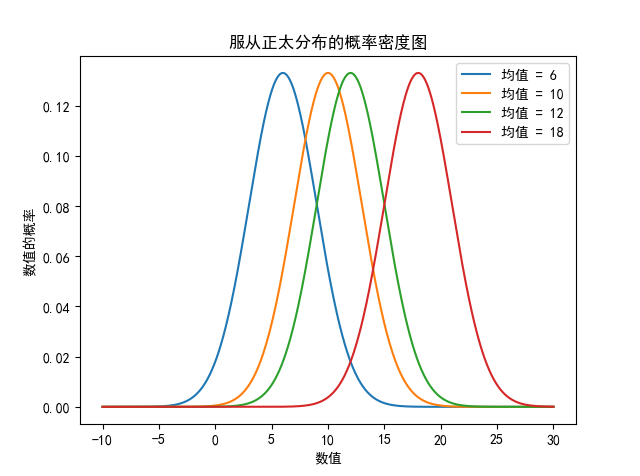由图像看出, 当标准差相同时(代码设置标准差相同), 峰值相同, 均值不同时, 对称轴位置不同
再次修改代码对标准差和均值重新赋值(可跳过不用看代码, 直接看图像)
import matplotlib.pyplot as plt
import math
import numpy as np
import matplotlib

matplotlib.rcParams['axes.unicode_minus']=False#解决保存图像时负号'-'显示为方块的问题
plt.rcParams['font.sans-serif'] = ['SimHei'] # 指定默认字体

x = np.linspace(-10,30,num=1000)  # x轴的取值范围
std1 = 3 # 定义标准差, 并输入标准差
mean1 = 6  # 定义均值,并输入均值
fx1 = 1 / (std1 * pow(2 * math.pi, 0.5)) * np.exp(-((x - mean1) ** 2) / (2 * std1 ** 2))  # 概率密度函数公式

std2 = 5
mean2 = 10
fx2 = 1 / (std2 * pow(2 * math.pi, 0.5)) * np.exp(-((x - mean2) ** 2) / (2 * std2 ** 2))  # 概率密度函数公式

std3 = 6
mean3= 12
fx3 = 1 / (std3 * pow(2 * math.pi, 0.5)) * np.exp(-((x - mean3) ** 2) / (2 * std3 ** 2))  # 概率密度函数公式

std4 = 2
mean4 = 18
fx4 = 1 / (std4 * pow(2 * math.pi, 0.5)) * np.exp(-((x - mean4) ** 2) / (2 * std4 ** 2))  # 概率密度函数公式

# 多条曲线在同一张图上进行对比
plt.plot(x, fx1,label = '均值 = 6, 标准差=3')  # 绘制概率密度函数图像
plt.plot(x,fx2,label = '均值 = 10, 标准差=5')
plt.plot(x,fx3,label = '均值 = 12, 标准差=6')
plt.plot(x,fx4,label = '均值 = 18, 标准差=2')
plt.legend() # 显示标签 label
plt.xlabel("数值")
plt.ylabel('数值的概率')
plt.title('服从正太分布的概率密度图')
plt.show()  # 显示图像

图像显示: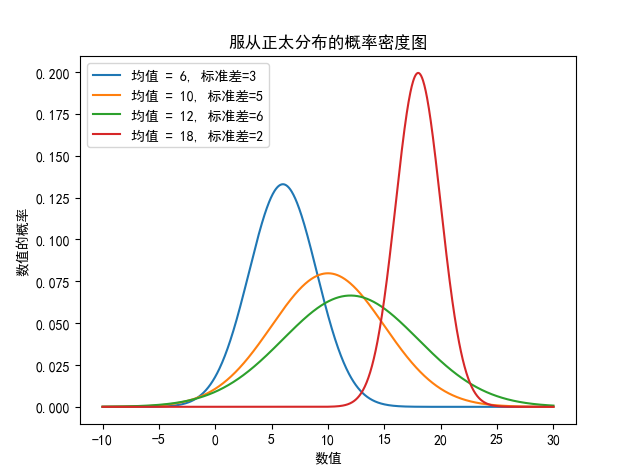通过图像可以观查出均值和标准差对概率的影响, 验证了上述的结论
最后: 本人又另写一篇关于服从正太分布概率 离散情况下 概率密度函数和累积密度函数的文章. 有疑惑的可以查看一下
展开全文正态分布
• 概率密度函数 分布概率密度函数（PDF）。 随机变量的（PDF）为 其中alpha是形状参数， beta是速率参数。 安装 $npm install distributions-gamma-pdf 要在浏览器中使用，请使用 。 用法 var pdf = require ( '...JavaScript • 概率密度函数非参数估计matlab代码使用Python进行均值平移分割 执行均值平移分割以跟踪图像序列上的对象。 均值漂移细分实施者：Agam Deep Arora（50169805）和Debika Dutt（50170009）提交日期：15年12月14日2.文献... • 2）python绘制正态分布的概率密度函数图象 4、卡方分布的概率密度函数及其图象 1）卡方分布的概率密度函数及其图象 2）python绘制卡方分布的概率密度函数图象 5、t分布的概率密度... • 概率密度函数 分布概率密度函数（PDF）。 随机变量的（PDF）为 其中sigma是比例参数。 安装$ npm install distributions-rayleigh-pdf 要在浏览器中使用，请使用 。 用法 var pdf = require ( 'distributions-...JavaScript
• Python计算一组数据的PDF（概率密度函数）方法 公式如下： python实现： 第一种方法： import scipy.stats as st st.norm.pdf([一组数据]) 第二种方法： def pdf(x, mean, var): return exp(-(x - mean) ** 2 / ...
Python计算一组数据的PDF（概率密度函数）方法
公式如下：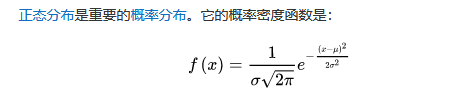python实现：
第一种方法：
import scipy.stats as st

st.norm.pdf([一组数据])

第二种方法：
def pdf(x, mean, var):
return exp(-(x - mean) ** 2 / (2 * var ** 2)) / sqrt(2 * pi) * var

如果是对dataframe进行操作可以抽取单列用拉姆达函数：
df['R_pdf'] = df['Rencency'].apply(lambda x: pdf(x, R_avg, R_std))

如果是进一步计算CDF累计概率密度函数，可以对PDF进行积分，或者用
import scipy.stats as st

st.norm.cdf([一组数据])

展开全文数据分析 数据挖掘
• 高斯分布以及概率密度函数Python）（持续更新）前言一、高斯分布二、概率密度函数 前言 项目上需要用到高斯分布、正太分布以及概率密度函数。我对这部分知识点产生了一些疑问，上网查资料发现很少有人在我这个点...


高斯分布以及概率密度函数（Python）（持续更新）
前言一、高斯分布二、概率密度函数

前言
项目上需要用到高斯分布、正太分布以及概率密度函数。我对这部分知识点产生了一些疑问，上网查资料发现很少有人在我这个点产生疑问（？？？可能是我太蠢了吧哈哈哈哈哈），所以我整理了一下自己看一看，如果后续有补充的会不断更新。

一、高斯分布
高斯分布究竟是个啥东西，网上介绍的人都介绍烂了，这里就不再赘述了。这里展示一下它的函数：

f

(

x

)

=

1

2

Π

σ

2

e

−

(

x

−

μ

)

2

2

σ

2

f(x) = \frac{1}{\sqrt{2Πσ^2}}e^{-\frac{(x-μ)^2}{2σ^2}}

二、概率密度函数
就是上一节展示的函数。。。
然后呢我的项目是这样（mean和σ的数值如图所示）：我们想要求

x

=

2.06

x=2.06

的概率，当然我们知道的是一点的概率为零，这里就要用到概率密度函数了。概率等于：

f

(

x

)

=

1

2

Π

σ

2

e

−

(

x

−

μ

)

2

2

σ

2

f(x) = \frac{1}{\sqrt{2Πσ^2}}e^{-\frac{(x-μ)^2}{2σ^2}}

P

=

f

(

x

)

∗

s

i

g

m

a

P = f(x) * sigma就这样，写完了，有更新的内容我再回来补充，先这样，各位再见。
展开全文• 我让Python返回10000个参数为n和p的二项式随机变量 进行10000次实验，每次抛10次硬币，统计有几次正面朝上，最后统计每次实验正面朝上的次数 ''' binom_sim = stats.binom.rvs(n=10,p=0.3,size=10000) print(len...
• import matplotlib.pyplot as plt import numpy as np from scipy import stats from matplotlib import style style.use('fivethirtyeight') mu_params = [-1, 0, 1] sd_params = [0.5, 1, 1.5] ...
• mumpy 、random import numpy as np import seaborn as sns import matplotlib.pyplot as plt from scipy import stats np.random.seed(1234) rn1 = np.random.normal(loc = 0, scale = 1, size = 1000) ...
• python 服从正太分布 离散情况 下 的概率密度函数 scipy.stats库是分布函数库, 包含正太分布,泊松分布,卡方分布等 导入scipy.stats库以后, 应用函数norm生成服从正太分布的概率密度函数, 具体法是: scipy...norm
• 计算分布函数(也称累积概率函数CDF) python代码如下： import numpy as np data = np.random.randn(200) # 随机生成一些数 # 一个公式即可计算出概率函数 cdf_value = 1. * np.arange(len(data)) / (len(data) - 1...
• # python 服从正太分布下概率密度函数 利用input()函数输入均值和标准差, 多次绘制概率密度函数图形并将图像曲线放置在同一张图中 代码块: """ 绘制正太分布函数曲线图 """ import matplotlib.pyplot as plt import...循环输入 正太分布
• 实现的方法可以不止一种： ...本篇介绍根据累积概率分布函数的逆函数(2：invert the CDF)生成的方法。 原参考网站：https://blog.demofox.org/2017/08/05/generating-random-numbers-from-a-speci...随机数
• 二维高斯分布概率密度函数数据集实战优化坐标轴与图像优化图像再次优化 概率密度函数 大家肯定都有听说过正态分布，其实正态分布只是概率密度分布的一种，正态分布的概率密度函数均值为μ ，标准差σ是高斯函数的一...数据分析 机器学习
• ## 使用Python估计数据概率分布函数

万次阅读 多人点赞 2016-11-23 16:10:47
首先其文档对我这种六级低空飘过的人来说读着好烦恼，所以，对其参数设置什么的不是很理解，从而导致得到的pdf(概率密度函数)效果不是很好。 Plan B 继续使用Seaborn模块 后来我转念一想，seaborn返回的图中...数据
• 我们在统计数据处理时，经常计算一个样本的概率密度估计，也就是说给出一组统计数据，要求你绘制出它的概率分布曲线，matlab的统计工具箱中有直接的函数就是：Ksdensity核心平滑密度估计 [f,xi] = ksdensity(x) ...
• 1.概率密度函数 　1.1. 定义 　如果对于随机变量X的分布函数F(x),存在非负函数f(x),使得对于任意实数有 　则称X为连续型随机变量，其中F(x)称为X的概率密度函数，简称概率密度。(f(x)>=0,若f(x)在点x处连续则F...机器学习 算法 人工智能 html
• 文章目录术语前言整数浮点数抽取字节洗牌排列贝塔分布二项分布卡方分布狄利克雷分布指数分布F分布伽玛分布几何分布耿贝尔分布超几何分布拉普拉斯分布（双指数分布）逻辑斯谛分布正态...pdf，概率密度函数（Probability
• 概率密度函数非参数估计matlab代码代码-Matlab 2017a / Python 3.7-MSAL（多标准优化主动学习）算法 主动学习选择最关键的实例，并通过与Oracle的交互来获取它们的标签。 选择信息量大或代表性的未标记实例可能会...
• beta分布的最大特点是其多样性, 从下图可以看出, beta分布具有各种形态, 有U形, 类似正态分布的形状, 类似uniform分布的形状等, 正式这一特质使beta分布在共轭先验的计算中起到重要作用: import matplotlib.pyplot ......

# python求概率密度函数python 订阅